• 哈工大形式语言与自动机历年试题，含答案的哦，仅供参考
• 我是哈工大的学长，特此为学弟们准备了哈尔滨工业大学形式语言作业-综合楼-自动机，本作业是与综合楼相关的，内容详实，可供参考。
• 哈尔滨工业大学形式语言讲义，适合有一定数学基础，是计算机学科必不可少的数学技能。
• 哈工大计算学部2019级，春雨老师班，资料是2019，2020c的试卷以及自己写的答案（不保证正确性），以及自己总结的知识点，最重要的：春雨gg画的重点。也可以在...
• 哈工大形式语言与自动机部分历年试题，含答案，仅供参考，祝考试成功哈
• 形式语言哈工大计算机特色科目。课件自然很是珍贵。
• 哈尔滨工业大学2019年《形式语言与自动机》期末试题 Design a DFA for the language L = {w∈{0,1}* | w contains both 01 and 10 as substrings}. Design a NFA within four states for the language {a}*∪{ab...
哈尔滨工业大学2019年《形式语言与自动机》期末试题
Design a DFA for the language L = {w∈{0,1}* | w contains both 01 and 10 as substrings}.  Design a NFA within four states for the language {a}*∪{ab}*.  Design regular expressions for language over Σ = {0,1}. (1).All strings contain the substring 001. (2).All strings expect the string 001.  Prove that L = {0m1n | m/n is an integer} is not regular with pumping lemma.  Convert the following NFA into DFA with subset construction.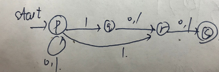Give a context-free grammar for L = { aibjci+j|i,j>=0}  Let L be the language generated by the grammar G below S->AB|BBB A->Bb|ε B->aB|A (1).消除空产生式 (2).消除单元产生式 (3).转换到CNF  Design a PDA for L = {w∈{a,b}*|w has more a’s than b’s}  Prove : for every context free language L, the language L’ = {0|w||w∈L} is also context free.  Design a Turing Machine that computes the following function f:0n->Binary(n) Where integer n>=1 and binary(n) is the binary representation of n. For example: f(03) = 11 f(05) = 101.
注：此题目为考试试卷实录，敬请放心食用。
展开全文期末考试
• 哈工大2020春形式语言与自动机期末试题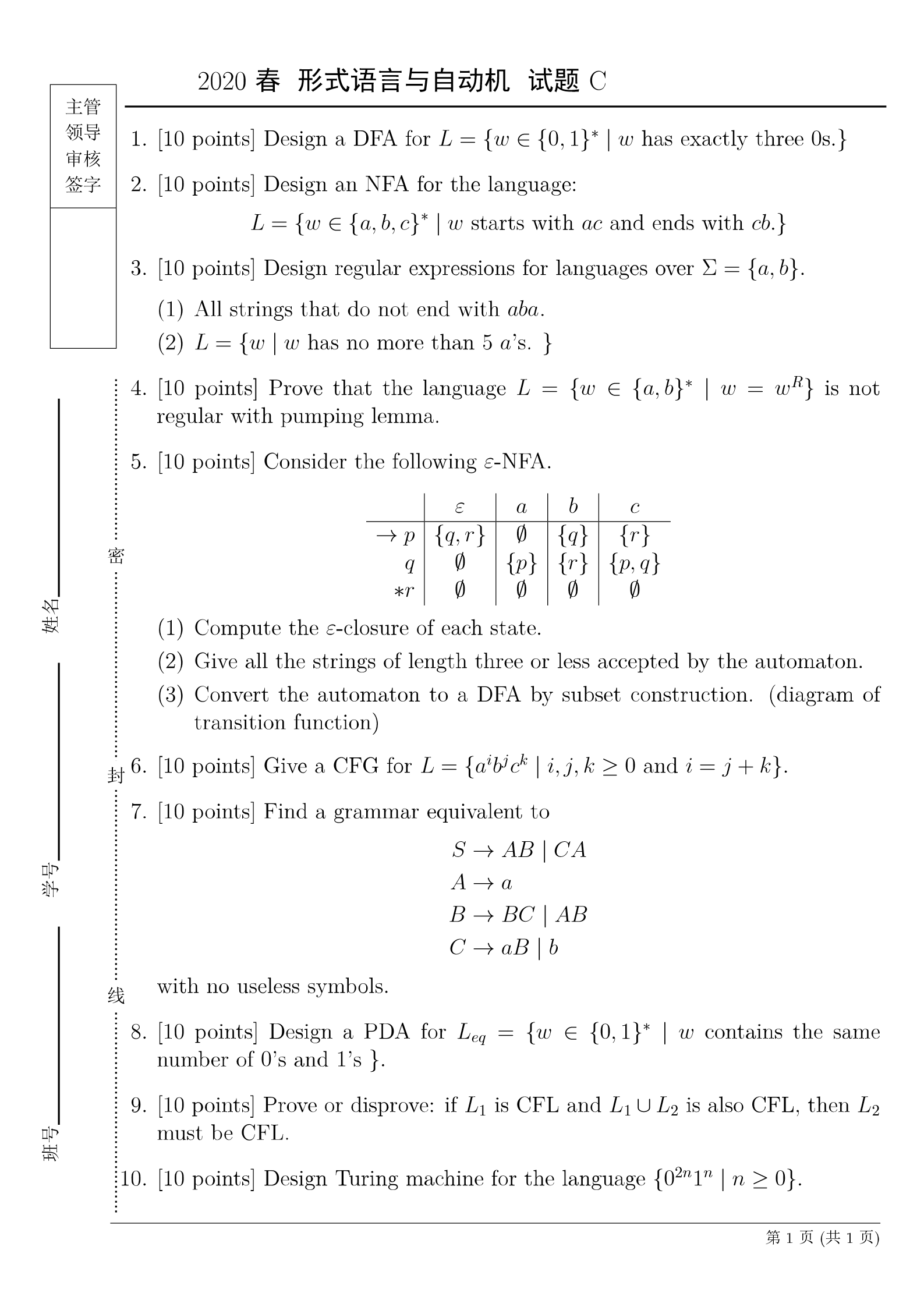展开全文确定性有穷自动机 图灵机 下推自动机
• 形式语言与自动机 课程论文 是孙大烈老师教的 哈工大 计算机学院 10级 这个不是电梯 这是自选的论文
• 西苑宾馆电梯 形式语言与自动机 大作业 哈工大 孙大烈老师
• 1.为语言 L （ L over {0，1} ），设计一个PDA，L = {w | w end with 011} 2. 在五个状态内，为语言L 设计一个NFA，L={ w | w contains the substring 1011 } 3. 为 L 设计正则表达式，L over {a，b} 1） L={w| w ...
1.为语言 L （ L over {0，1} ），设计一个PDA，L = {w | w end with 011} 2. 在五个状态内，为语言L 设计一个NFA，L={ w | w contains the substring 1011 } 3. 为 L 设计正则表达式，L over {a，b} 1） L={w| w contains at most three a‘s} 2） L ={w | w doesn’t end with double letter } eg : aa , bb 4. 用泵引理证明 a^n b^m ( n = 2m) 不是正则的 5. 6. 晚点再更。。。。试卷是全英文的，懒得抄全，就中文表达题目意思
展开全文• 自动机理论正则表达式第一部 哈工大的东西本不外传的，要点分而已
• 自动机理论正则表达式第二部 哈工大的东西本不外传的，要点分而已
• 就是一篇很普通的形式语言与自动机的复习时的笔记而已 本文原载于我的博客，地址：https://blog.guoziyang.top/archives/21/
本文原载于我的博客，地址：https://blog.guoziyang.top/archives/21/
1 课程简介与基础知识
1.1 课程简介
自动机理论：图灵机、有限状态机、文法，下推自动机
形式语言：经数学定义的语言
课程内容：
正则语言：有穷自动机、正则表达式、正则语言的性质上下文无关语言：上下文无关文法、下推自动机、上下文无关语言的性质计算导论：图灵机及其拓展、不可判定性

1.2 基础知识
字母表：符号（或字符）的非空有穷集。
字符串：由某字母表中符号组成的有穷序列。
空串：记为

ϵ

\epsilon

，有0个字符的串。
字母表可以是任意的，但是都有

ϵ

∉

Σ

\epsilon\not\in\Sigma

。
字符串的长度：字符串中符号所占位置的个数，递归定义为 KaTeX parse error: No such environment: equation at position 8: \begin{̲e̲q̲u̲a̲t̲i̲o̲n̲}̲|\omega|=\begin… 字符串的连接：将首尾相接得到新字符串的运算，记为

x

⋅

y

x\cdot y

或者xy。
字符串的幂：字符串x的n次幂（n$\ge$0），递归定义为 KaTeX parse error: No such environment: equation at position 8: \begin{̲e̲q̲u̲a̲t̲i̲o̲n̲}̲x^n=\begin{case…
字符串集合的连接：得到字符串集合，将两个集合中的串两两连接。
集合的幂：

A

n

=

A

n

−

1

A

A^n=A^{n-1}A

克林闭包：

Σ

∗

=

∪

i

=

0

∞

Σ

i

\Sigma^*=\cup_{i=0}^{\infty}\Sigma^i

正闭包：

Σ

+

=

∪

i

=

1

∞

Σ

i

\Sigma^+=\cup_{i=1}^{\infty}\Sigma^i

与克林闭包相差一个

ϵ

\epsilon

语言：若

Σ

\Sigma

为字母表且

∀

L

⊂

Σ

∗

\forall L\subset\Sigma^*

，则L称为字母表

Σ

\Sigma

上的语言。

∅

,

{

ϵ

}

,

Σ

∗

\emptyset,\{\epsilon\},\Sigma^*

分别都是任意字母表

Σ

\Sigma

上的语言。
2 有穷自动机
2.2 确定的有穷自动机
[外链图片转存失败,源站可能有防盗链机制,建议将图片保存下来直接上传(img-CCx5SaZ9-1591105349225)(https://i.loli.net/2019/06/13/5d01bbc9d91a237478.png)]
确定的有穷自动机（DFA）：描述为A，A为一个五元组

A

=

(

Q

,

Σ

,

δ

,

q

0

,

F

)

A=(Q,\Sigma,\delta,q_0,F)

Q：有穷状态集

Σ

\Sigma

：有穷输入符号集或字母表

δ

\delta

：

Q

×

Σ

→

Q

Q\times\Sigma\rightarrow Q

，状态转移函数

q

0

∈

Q

q_0\in Q

：初始状态

F

⊂

Q

F\subset Q

：终结状态集或接收状态集
一种描述：状态转移表：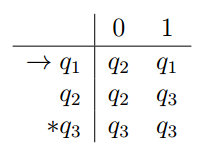扩展转移函数

δ

^

\hat{\delta}

：

Q

×

Σ

∗

→

Q

Q\times\Sigma^*\rightarrow Q

： KaTeX parse error: No such environment: equation at position 8: \begin{̲e̲q̲u̲a̲t̲i̲o̲n̲}̲\hat{\delta}(q,… 举例：（有误）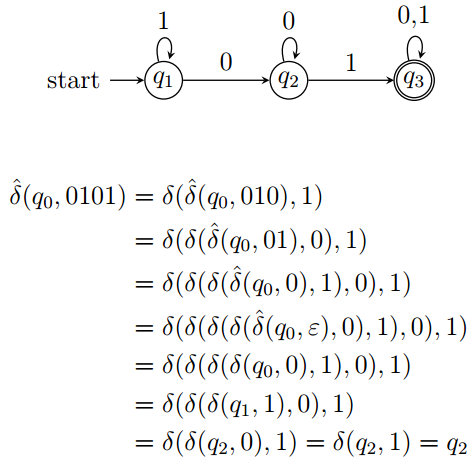DFA的语言与正则语言：
若 D = (Q, Σ, δ, q0, F) 是一个DFA, 则 D 接受的语言为

L

(

D

)

=

{

ω

∈

Σ

∗

∣

δ

^

(

q

0

,

ω

)

∈

F

}

L(D)=\{\omega\in\Sigma^*|\hat{\delta}(q_0,\omega)\in F\}

如果语言 L 是某个 DFA D 的语言, 即 L = L(D), 则称 L 是正则语言。
2.3 非确定有穷自动机
形式定义 ：
非确定有穷自动机（NFA）：

A

=

(

Q

,

Σ

,

δ

,

q

0

,

F

)

A=(Q,\Sigma,\delta,q_0,F)

Q：有穷状态集

Σ

\Sigma

：有穷输入符号集或字母表

δ

\delta

：

Q

×

Σ

→

2

Q

Q\times\Sigma\rightarrow 2^Q

，状态转移函数，即可以转移到多种状态

q

0

∈

Q

q_0\in Q

：初始状态

F

⊂

Q

F\subset Q

：终结状态集或接收状态集
扩展转移函数

δ

^

\hat{\delta}

：

Q

×

Σ

∗

→

2

Q

Q\times\Sigma^*\rightarrow 2^Q

： KaTeX parse error: No such environment: equation at position 8: \begin{̲e̲q̲u̲a̲t̲i̲o̲n̲}̲\hat{\delta(q, … 举例：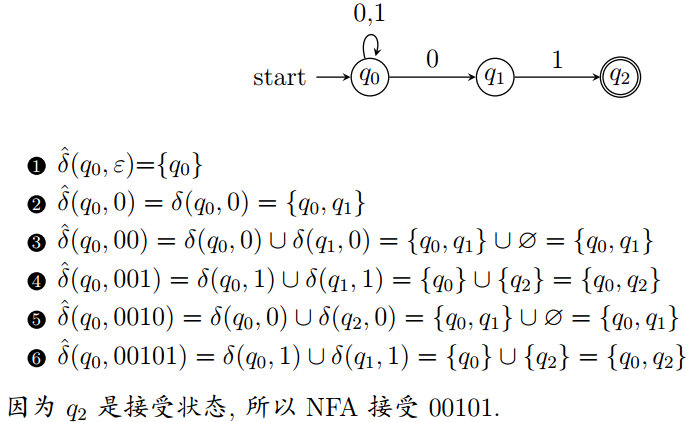DFA与NFA的等价性：
如果语言L被NFA接受，当且仅当L被DFA接受。
子集构造法（构造与NFA等价的DFA）：如果 NFA N=

(

Q

N

,

Σ

,

δ

N

,

q

0

,

F

N

)

(Q_N, \Sigma, \delta_N, q_0, F_N)

构造 DFA D=

(

Q

D

,

Σ

,

δ

D

,

{

q

0

}

,

F

D

)

(Q_D,\Sigma,\delta_D,\{q_0\}, F_D)

Q

D

=

2

Q

N

Q_D=2^{Q_N}

F

D

=

{

S

∣

S

⊂

Q

N

,

S

∩

F

N

≠

∅

}

F_D=\{S|S\subset Q_N,S\cap F_N\not=\emptyset\}

∀

S

⊂

Q

N

,

∀

a

∈

Σ

,

δ

D

(

S

,

a

)

=

∪

p

∈

S

δ

N

(

p

,

a

)

\forall S\subset Q_N,\forall a\in\Sigma,\delta_D(S, a)=\cup_{p\in S}\delta_N(p,a)

则有L(D)=L(N)
举例：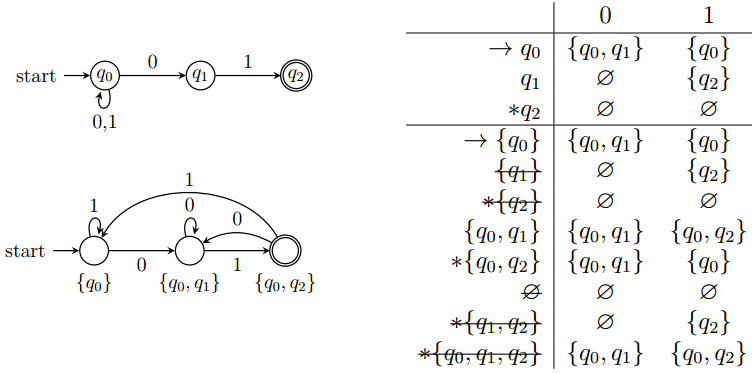2.4 带空转移的非确定有穷自动机
允许状态因空串

ϵ

\epsilon

而转移, 即不消耗输入字符就发生状态的改变。
带空转移非确定有穷自动机(

ϵ

\epsilon

-NFA)：

A

=

(

Q

,

Σ

,

δ

,

q

0

,

F

)

A=(Q,\Sigma,\delta,q_0,F)

Q：有穷状态集

Σ

\Sigma

：有穷输入符号集或字母表

δ

\delta

：

Q

×

(

Σ

∪

{

ϵ

}

)

→

2

Q

Q\times(\Sigma\cup\{\epsilon\})\rightarrow 2^Q

，状态转移函数

q

0

∈

Q

q_0\in Q

：初始状态

F

⊂

Q

F\subset Q

：终结状态集或接收状态集
状态的

ϵ

\epsilon

-闭包：记为

E

C

L

O

S

E

(

q

)

E_{CLOSE}(q)

，表示从q经过

ϵ

\epsilon

序列
可达的全部状态集合。
状态集S的

ϵ

−

\epsilon-

闭包：

E

C

L

O

S

E

(

S

)

=

∪

q

∈

S

E

C

L

O

S

E

(

q

)

E_{CLOSE}(S)=\cup_{q\in S}E_{CLOSE}(q)

。
扩展转移函数

δ

^

\hat{\delta}

：

Q

×

Σ

∗

→

2

Q

Q\times\Sigma^*\rightarrow 2^Q

： KaTeX parse error: No such environment: equation at position 8: \begin{̲e̲q̲u̲a̲t̲i̲o̲n̲}̲\hat{\delta}(q,… 举例：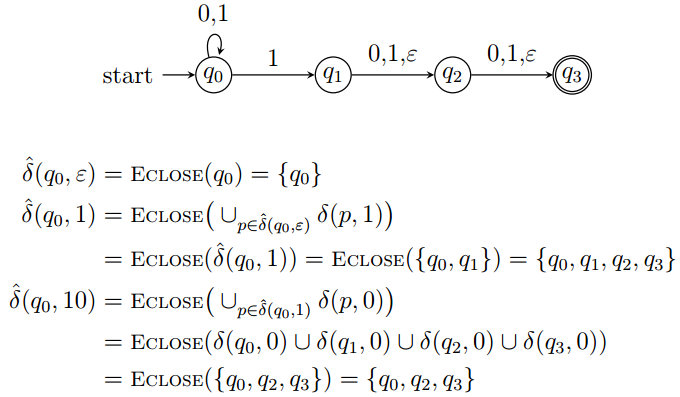ϵ

\epsilon

-NFA的语言：若E=(

Q

,

Σ

,

δ

,

q

0

,

F

Q,\Sigma,\delta, q_0,F

)是一个

ϵ

−

N

F

A

\epsilon-NFA

，则E接受的语言为

L

(

F

)

=

{

ω

∈

Σ

∗

∣

δ

^

(

q

0

,

ω

)

∩

F

≠

∅

}

L(F)=\{\omega\in\Sigma^*|\hat{\delta}(q_0,\omega)\cap F\not=\emptyset\}

消除空转移的子集构造法：
如果

ϵ

−

N

F

A

E

=

(

Q

E

,

Σ

,

δ

E

,

q

E

,

F

E

)

\epsilon-NFA E=(Q_E,\Sigma,\delta_E,q_E,F_E)

，构造DFA：

D

=

(

Q

D

,

Σ

,

δ

D

,

q

D

,

F

D

)

D=(Q_D,\Sigma,\delta_D,q_D,F_D)

Q

D

=

2

Q

E

Q_D=2^{Q_E}

，或

Q

D

=

{

S

⊂

Q

E

∣

S

=

E

C

L

O

S

E

(

S

)

}

Q_D=\{S\subset Q_E|S=E_{CLOSE}(S)\}

。

q

D

=

E

C

L

O

S

E

(

q

E

)

q_D=E_{CLOSE}(q_E)

。

F

D

=

{

S

∣

S

∈

Q

D

,

S

∩

F

E

≠

∅

}

F_D=\{S|S\in Q_D,S\cap F_E\not=\emptyset\}

。

∀

S

∈

Q

D

,

∀

a

∈

Σ

,

δ

D

(

S

,

a

)

=

E

C

L

O

S

E

(

∪

p

∈

S

δ

E

(

p

,

a

)

)

\forall S\in Q_D,\forall a\in\Sigma,\delta_D(S,a)=E_{CLOSE}(\cup_{p\in S}\delta_E(p,a))

。
那么有

L

(

D

)

=

L

(

E

)

L(D)=L(E)

。
举例：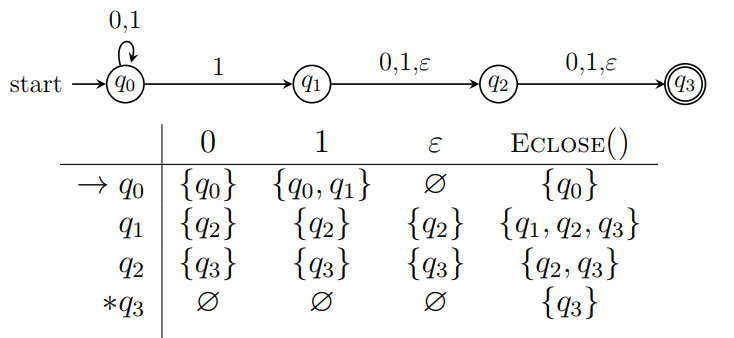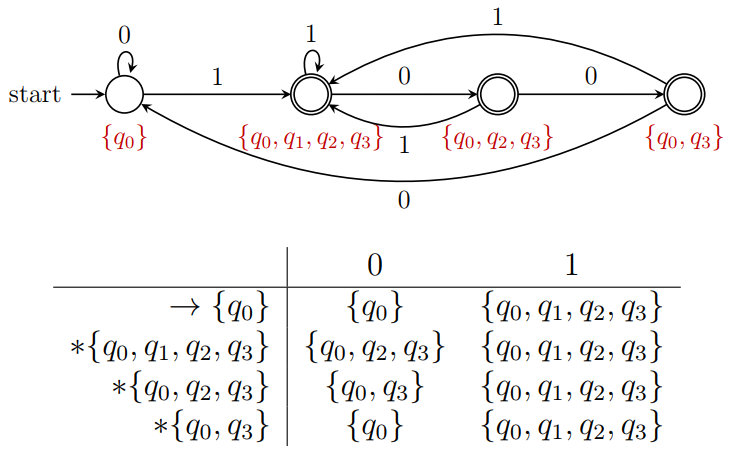定理：如果语言L被

ϵ

−

N

F

A

\epsilon-NFA

接受，当且仅当L被DFA接受。
3 正则表达式
3.1 正则表达式
有穷自动机通过机器装置描述正则语言
通过表达式描述正则语言
语言的运算：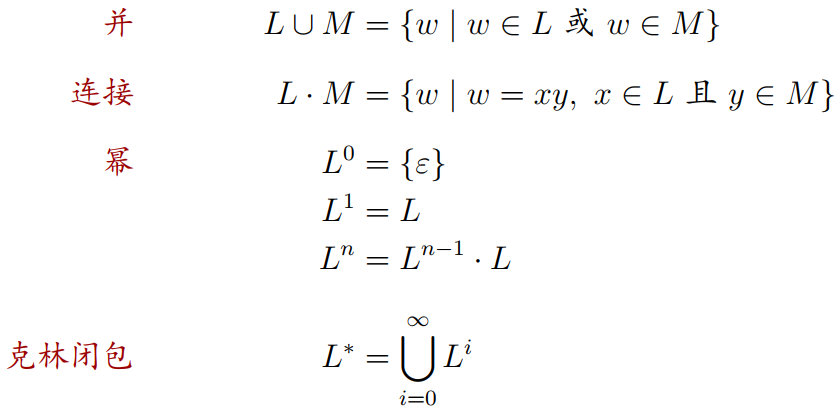注意：语言的幂将字符添加在已有字符串的后面。
四则运算表达式的递归定义：
任何数都是四则运算表达式如果a和b是四则运算表达式，那么

a

+

b

,

a

−

b

,

a

×

b

,

a

÷

b

a+b,a-b,a\times b,a\div b

和(a)都是四则运算表达式
正则表达式的递归定义：
如果

Σ

\Sigma

为字母表，则

Σ

\Sigma

上的正则表达式递归定义为：

∅

\emptyset

是一个正则表达式，表示空语言，

ϵ

\epsilon

是一个正则表达式，表示语言

{

ϵ

}

\{\epsilon\}

，

∀

a

∈

Σ

,

a

\forall a\in\Sigma,a

是一个正则表达式，表示语言

{

a

}

\{a\}

。如果正则表达式r和s分别表示语言R和S，那么

r

+

s

,

r

s

,

r

∗

,

(

r

)

r+s,rs,r^*,(r)

都是正则表达式，分别表示语言

R

∪

S

,

R

⋅

S

,

R

∗

,

R

R\cup S,R\cdot S,R^*,R

。
运算符优先级：
括号，星，连接（

r

s

,

r

⋅

s

rs,r\cdot s

），加（

r

+

s

,

r

∪

s

r+s,r\cup s

）
3.2 有穷自动机和正则表达式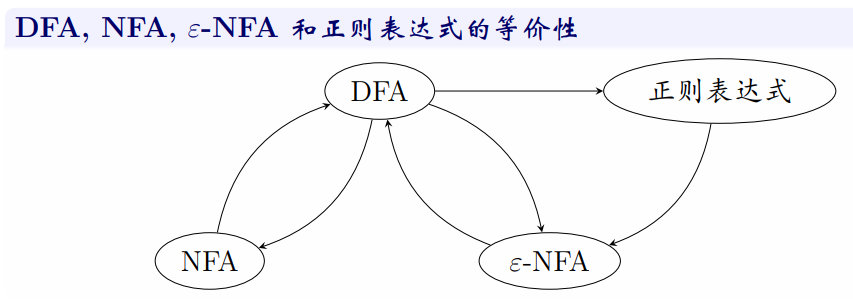**定理：**若L=L(A)是某DFA A的语言，那么存在正则表达式R满足L=L®。
将DFA转化为正则表达式（递归表达式法）：
正则表达式

R

i

j

(

k

)

R_{ij}^{(k)}

表示从i到j但中间节点不超过k全部路径的字符串集
举例：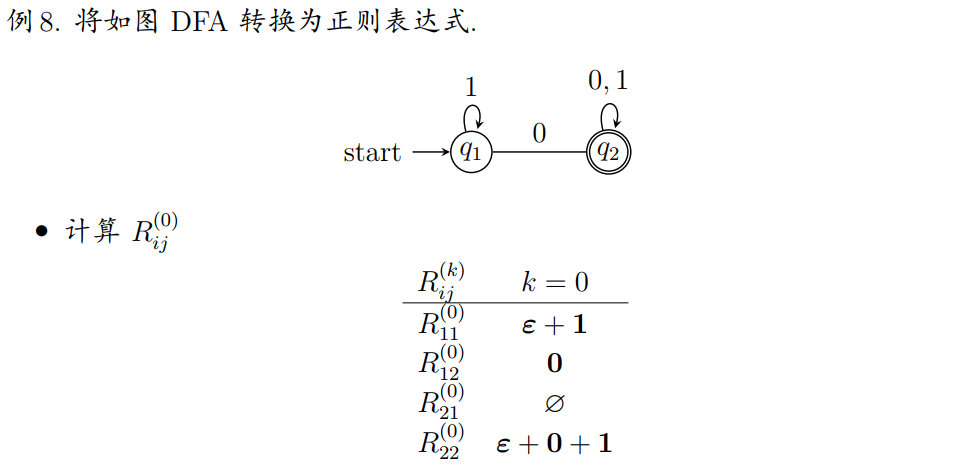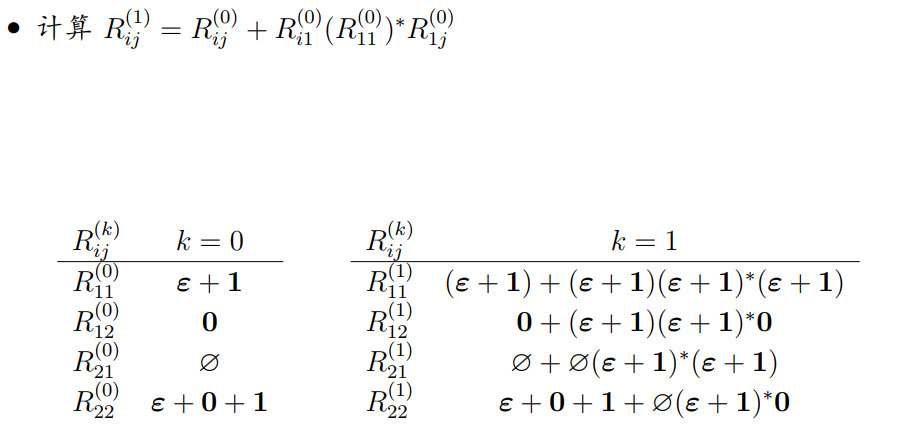注意：自己到自己包括

ϵ

\epsilon

化简规则：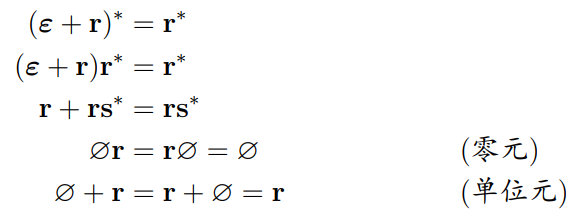继续上面的例子：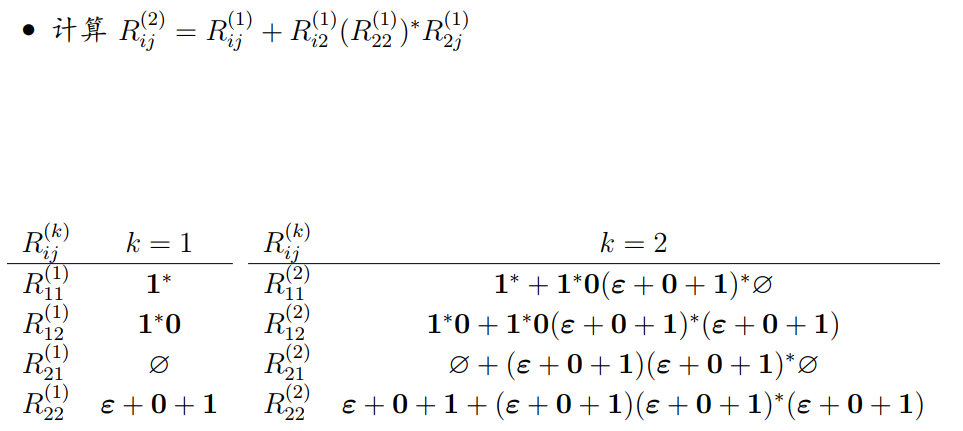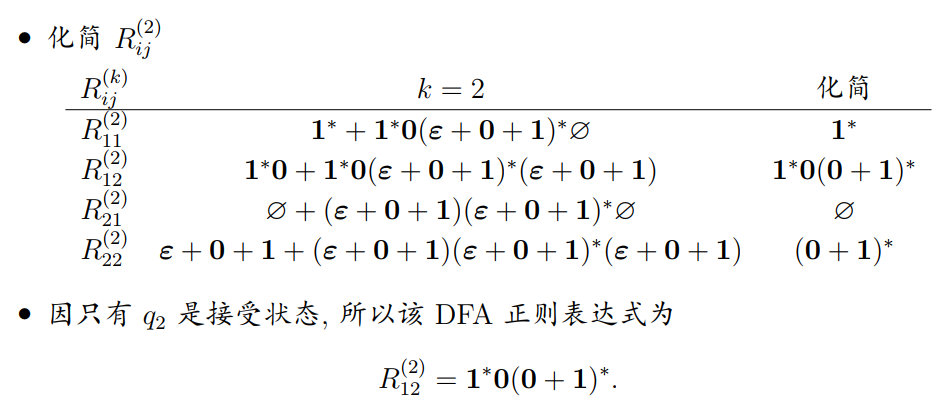DFA 到正则表达式（状态消除法）：
从DFA中逐个删除状态，保证"自动机"的等价。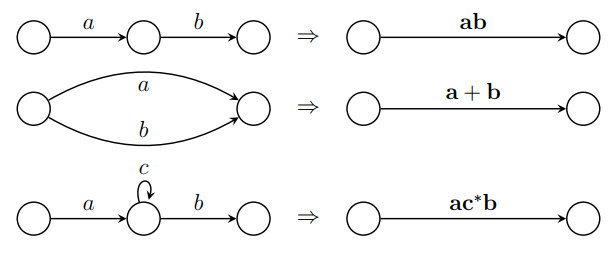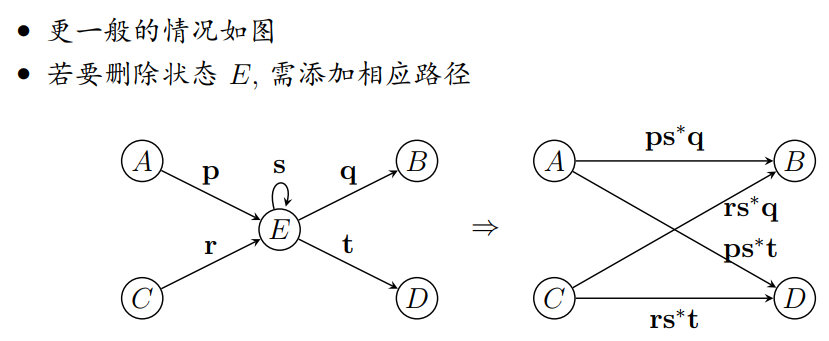注意：在使用状态消除法时，需要利用空转移添加开始状态s和结束状态f。
从正则表达式到有穷自动机：
正则表达式定义的语言，都可以被有穷自动机识别。
构造规则：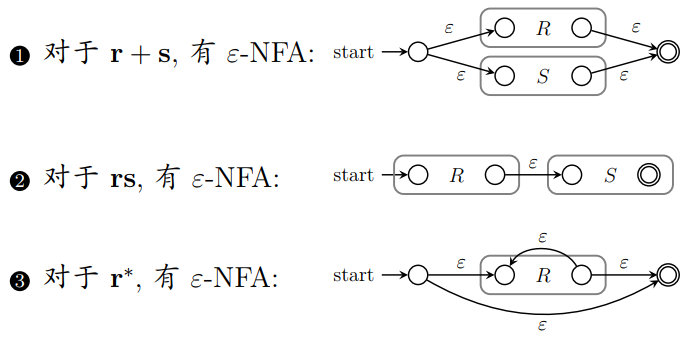3.3 正则表达式的代数定律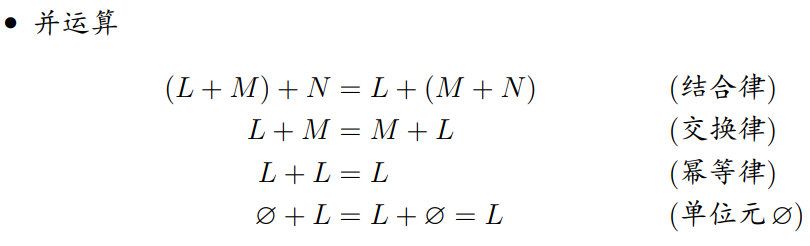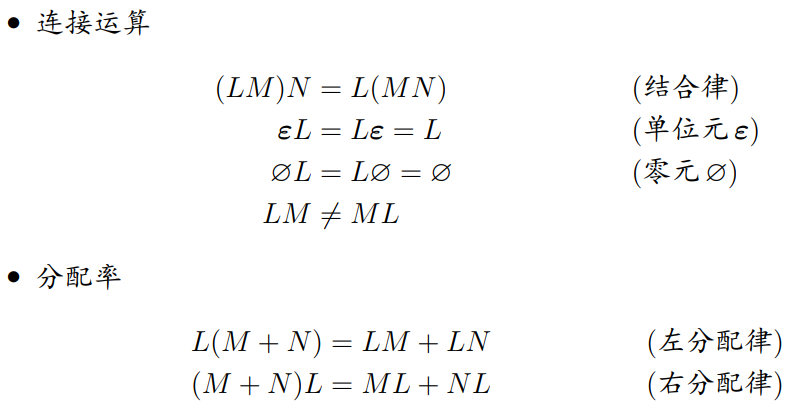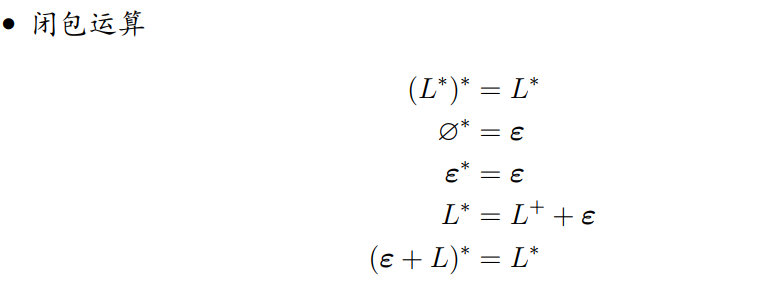4 正则语言的性质
4.1 证明语言的非正则性
正则语言的泵引理：
如果语言 L 是正则的，那么存在正整数N，它只依赖于L，对

∀

ω

∈

L

\forall\omega\in L

，只要

∣

ω

∣

≥

N

|\omega|\ge N

，就可以将

ω

\omega

分为三部分

ω

=

x

y

z

\omega=xyz

满足：

y

≠

ϵ

(

∣

y

∣

>

0

)

y\not=\epsilon(|y|>0)

∣

x

y

∣

≤

N

|xy|\le N

∀

k

≥

0

,

x

y

k

z

∈

L

\forall k\ge 0,xy^kz\in L

举例：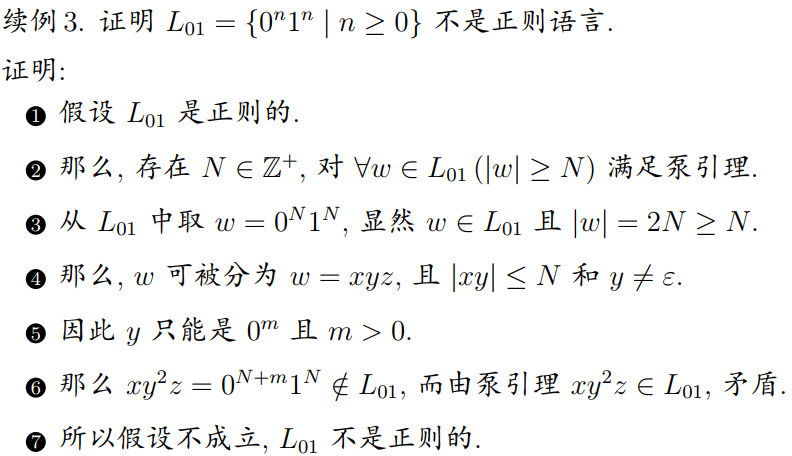若一个语言的一部分已经被证明不是正则的，则这个语言不是正则的。
注意：泵引理只是必要条件，只能用于证伪。
4.2 正则语言的封闭性
正则语言在经过以下运算后得到的仍然为正则语言，称为在这些运算下封闭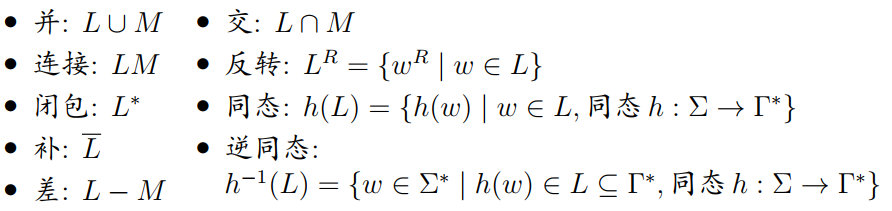求补运算：将所有的接受和非接受状态反转（注意这种方法需要完整的DFA）
可通过证明一个语言的补非正则，而证明该语言非正则
4.3 正则语言的判定性质
定理：具有n个状态的有穷自动机M接受的集合S：
S是非空的，当且仅当M接受某个小于n的串。S是无穷的，当且仅当M接受某个长度为m的串，

n

≤

m

≤

2

n

n\le m\le 2n

。
所以，对于正则语言：
存在算法, 判断其是否为空, 只需检查全部长度小于 n 的串。存在算法, 判断其是否无穷, 只需检查全部长度由 n 到 2n − 1 的串。
定理：存在算法判定两个有穷自动机是否等价（接受语言相同）
4.4 自动机的最小化
**定义：**DFA A=(

Q

,

Σ

,

δ

,

q

0

,

F

Q,\Sigma,\delta,q_0,F

)中的两个状态p和q，对

∀

ω

∈

Σ

∗

\forall\omega\in\Sigma^*

：

δ

^

(

p

,

ω

)

∈

F

⟷

δ

^

(

q

,

ω

)

∈

F

\hat{\delta}(p,\omega)\in F\longleftrightarrow\hat{\delta}(q,\omega)\in F

则称这两个状态是等价的，否则称为可区分的。
填表算法：如果填表算法不能区分两个状态，则这两个状态等价。
直接标记终态和非终态之间的状态对标记所有经过字符0到达终态和非终态的状态对标记所有经过字符1到达终态和非终态的状态对剩余的未标记状态对逐个检查，如果该状态对中的每个状态都可经过相同的串到达某可区分的状态对，则该状态对可区分而剩余的状态对经过很短的字符串后，都会到达相同状态，所以是等价的
举例：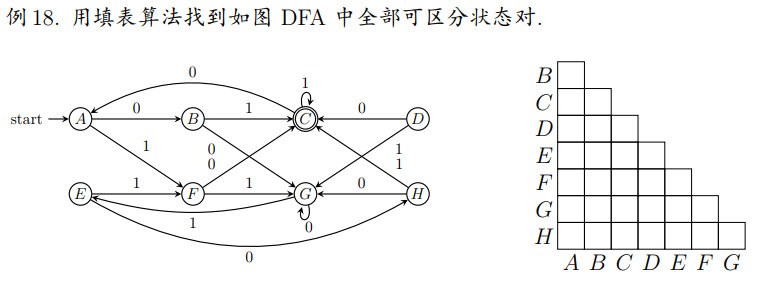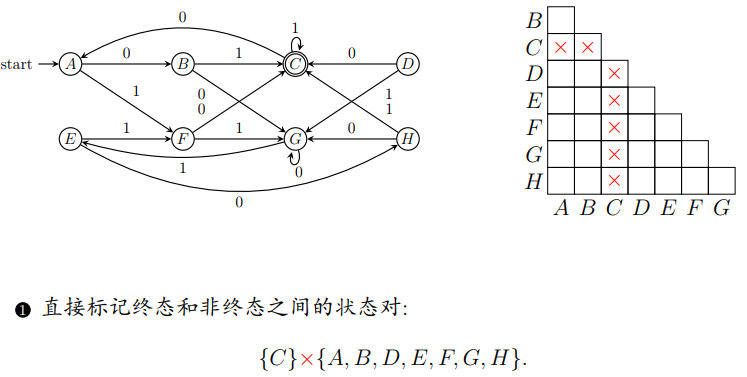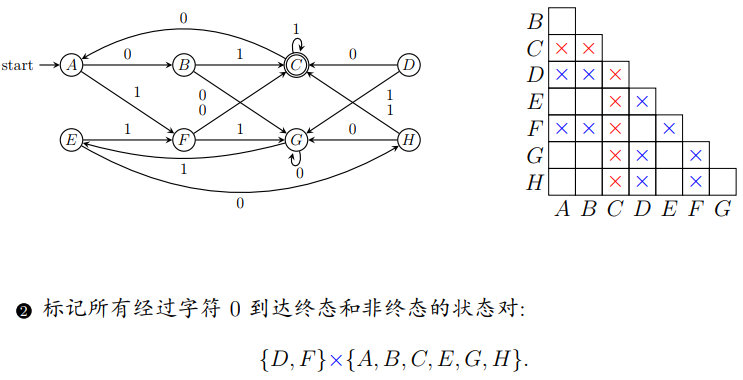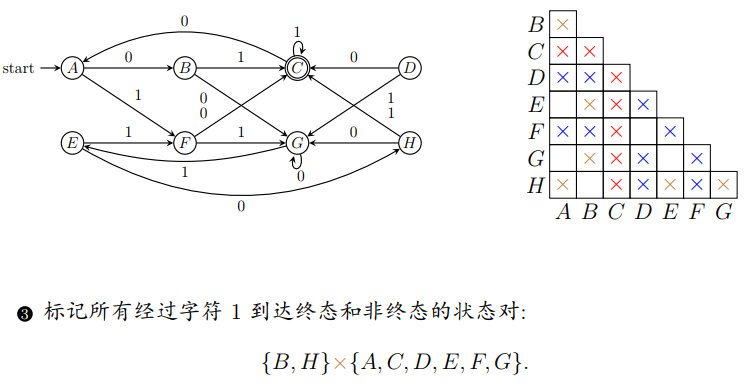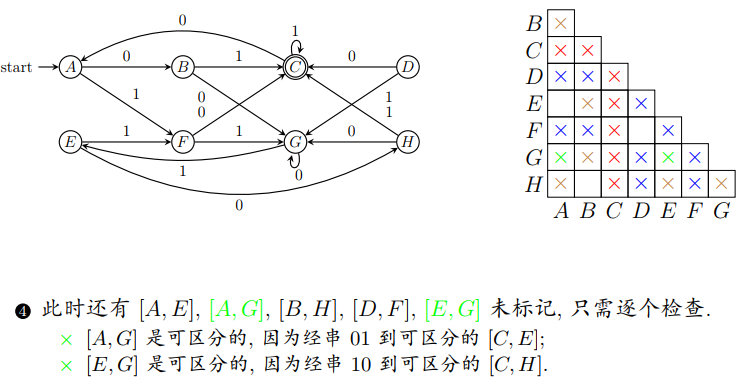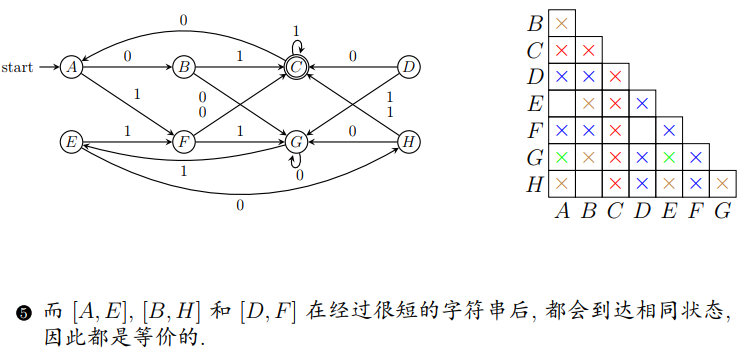最小化：根据等价状态，将状态集划分为块，合并等价状态即可
5 上下文无关文法
5.1 上下文无关文法
如果字符串

ω

∈

Σ

∗

\omega\in\Sigma^*

满足

ω

=

ω

R

\omega=\omega^R

，则称字符串

ω

\omega

为回文。如果语言L中的字符串都是回文，则称L为回文语言。
回文的递归表示：以

L

p

a

l

=

{

ω

∈

{

0

,

1

}

∗

∣

ω

=

ω

R

}

L_{pal}=\{\omega\in\{0,1\}^*|\omega=\omega^R\}

为例
首先

ϵ

,

0

,

1

\epsilon,0,1

都是回文如果

ω

\omega

是回文，那么

0

ω

0

0\omega 0

和

1

ω

1

1\omega 1

也是回文
嵌套定义：

A

→

ϵ

A

→

0

A

→

1

A

→

0

A

0

A

→

1

A

1

A\rightarrow\epsilon\\A\rightarrow 0\\A\rightarrow 1\\A\rightarrow 0A0\\A\rightarrow 1A1

上下文无关文法（CFG）：

G

=

(

V

,

T

,

P

,

S

)

G=(V,T,P,S)

V：变元的有穷集，变元也称为非终结符或语法范畴
T：终结符的有穷集, 且 V

∩

\cap

T = ∅
P：产生式的有穷集, 每个产生式包括：
一个变元, 称为产生式的头或左部。一个产生式符号

→

\rightarrow

, 读作定义为。一个

(

V

∪

T

)

∗

(V\cup T)^*

中的符号串, 称为体或右部。

S

∈

V

S\in V

：初始符号，文法开始的地方
字符使用的一般规定：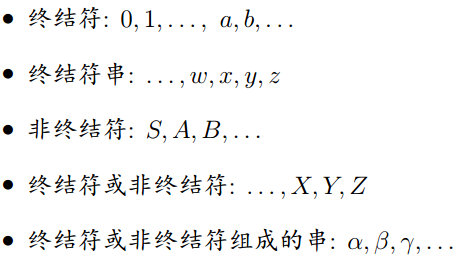从字符串到文法变元的过程，称为归约，逆过程称为派生。
举例：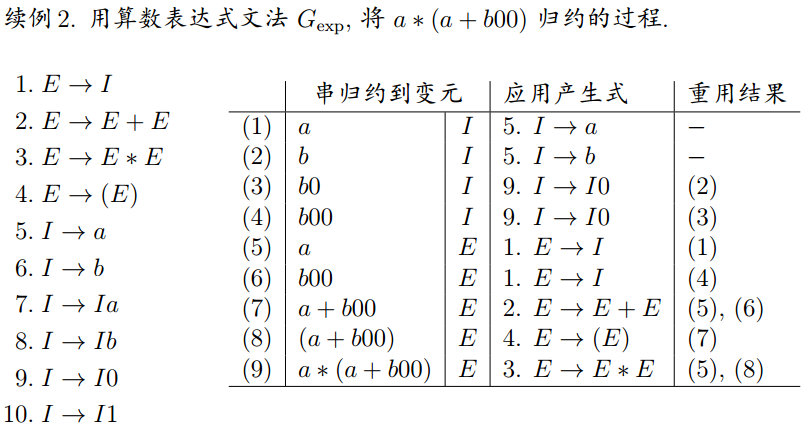派生和归约的形式定义：若CFG G=(V, T, P, S)，设

α

,

β

,

γ

∈

(

V

∪

T

)

∗

\alpha,\beta,\gamma\in(V\cup T)^*

，

A

∈

V

A\in V

，

A

→

γ

∈

P

A\rightarrow\gamma\in P

，那么称G中由

α

A

β

\alpha A\beta

可派生出

α

γ

β

\alpha\gamma\beta

，记为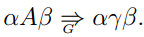相应的，称

α

γ

β

\alpha\gamma\beta

可归约为

α

A

β

\alpha A\beta

。
举例：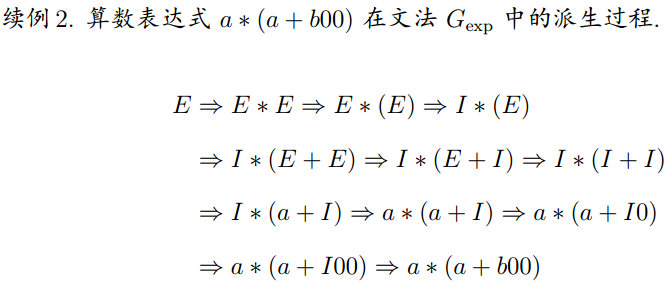最左派生：为限制派生的随意性, 要求只替换符号串中最左边变元的派生过程，称为最左派生。只替换最右的, 称为最右派生。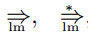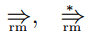任何派生都有等价的最左派生和最右派生。
文法的语言：CFG G=(V, T, P, S)的语言定义为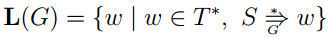那么符号串

ω

\omega

在L(G)中，要满足：

ω

\omega

仅由终结符组成初始符号S能派生出

ω

\omega

。
上下文无关语言定义：语言L是由某个CFG G 定义的语言，即 L = L(G)，则称 L 为上下文无关语言。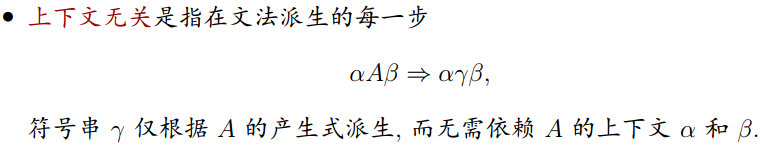如果有两个文法 CFG

G

1

G_1

和CFG

G

2

G_2

，满足

L

(

G

1

)

=

L

(

G

2

)

L(G_1)=L(G_2)

，则称

G

1

G_1

和

G

2

G_2

是等价的。
**句型：**若 CFG G = (V, T, P, S)，初始符号 S 派生出来的符号串，称为 G 的句型, 即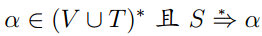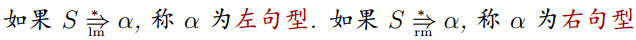只含有终结符的句型, 也称为 G 的句子而 L(G) 就是文法 G 全部的句子
所有的正则语言都是上下文无关语言。
5.2 语法分析树
派生或者归约的过程可以表示成树形结构
定义：CFG G = (V, T, P, S) 的语法分析树(语法树或派生树) 为
每个内节点标记为 V 中的变元符号每个叶节点标记为

V

∪

T

∪

{

ϵ

}

V\cup T\cup\{\epsilon\}

中的符号如果某内节点标记是 A, 其子节点从左至右分别为

X

1

,

X

2

,

…

,

X

n

X_1,X_2,…,X_n

，那么

A

→

X

1

,

X

2

,

…

,

X

n

∈

P

A\rightarrow X_1,X_2,…,X_n\in P

，若有

X

i

=

ϵ

X_i=\epsilon

，则

ϵ

\epsilon

是A唯一子节点，且

A

→

ϵ

∈

P

A\rightarrow\epsilon\in P

。
**定义：**语法树的全部叶结点从左到右连接起来，称为该树的产物或者结果
如果树根节点是初始符号 S，叶节点是终结符或 ε，那么该树的产物属于 L(G)。
语法树中标记为 A 的内节点及其全部子孙节点构成的子树，称为 A 子树。
语法分析树和派生的等价性：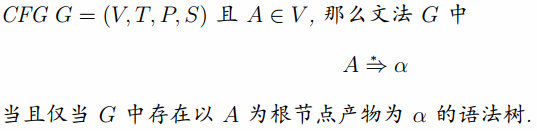语法树唯一确定最左 (右) 派生：
每棵语法分析树都有唯一的最左 (右) 派生，给定 CFG G = (V, T, P, S), A

∈

\in

V , 以下命题等价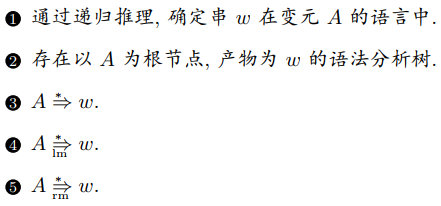5.3 文法和语言的歧义性
如果 CFG G 使某些符号串有两棵不同的语法分析树, 称文法 G 是歧义的。
例如：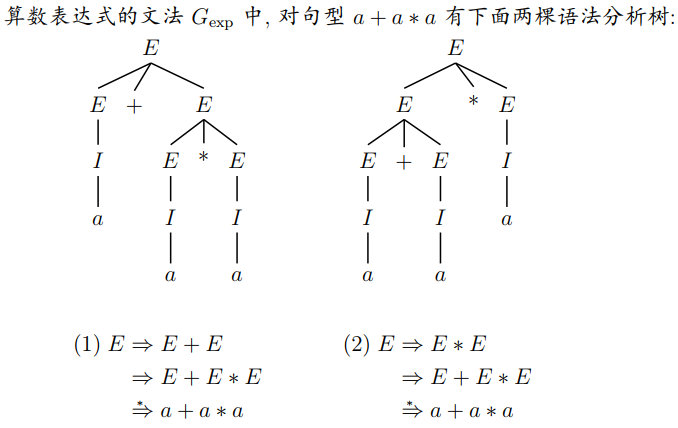可重新设计文法来消除歧义性
语言的固有歧义性：定义同样的语言可以有多个文法，如果 CFL L 的所有文法都是歧义的，那么称语言 L 是固有歧义的。
判断给定的语言是否歧义是一个不可判定的问题
5.4 文法的化简与范式
前提：不改变语言
步骤：
消除无用符号消除

ϵ

\epsilon

产生式：

A

→

ϵ

A\rightarrow\epsilon

消除单元产生式：

A

→

B

A\rightarrow B

无用符号：CFG G = (V, T, P, S), 符号

X

∈

(

V

∪

T

)

X\in(V\cup T)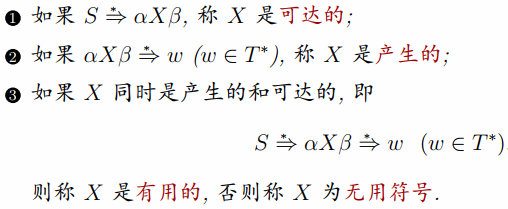消除无用符号：首先消除全部非产生的符号，再消除全部非可达的符号
产生的符号集：每个T中的符号（终结符）都是产生的，

A

→

α

∈

P

A\rightarrow\alpha\in P

且

α

\alpha

中符号都是产生的, 则A是产生的。（从终结符向前寻找）
可达的符号集：符号 S 是可达的，

A

→

α

∈

P

A\rightarrow\alpha\in P

且A是可达的，则

α

\alpha

中符号都是可达的。（从起始符向后寻找）
举例：消除如下文法的无用符号：

S

→

A

B

∣

α

，

A

→

b

S\rightarrow AB|\alpha，A\rightarrow b

：

先

消

除

非

产

生

的

：

S

→

α

A

→

b

再

消

除

非

可

达

的

：

S

→

a

先消除非产生的：\\S\rightarrow\alpha\\A\rightarrow b\\再消除非可达的：\\S\rightarrow a

消除

ϵ

−

\epsilon-

产生式：
文法中形如$A\rightarrow\epsilon 的 产 生 式 称 为 的产生式称为 \epsilon$-产生式
确定空可变元：
如果

A

→

ϵ

A\rightarrow\epsilon

, 则 A 是可空的如果$B\rightarrow\alpha 且 且 \alpha$中的每个符号都是可空的, 则 B 是可空的
替换产生式：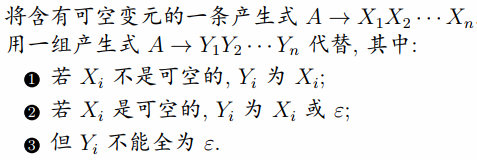消除单元产生式: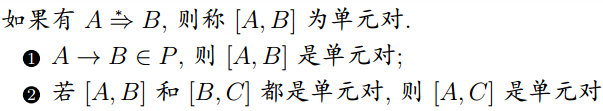消除单元产生式：
删除全部形为

A

→

B

A\rightarrow B

的单元产生式对每个单元对[A, B]，将B的产生式复制给A
定理：每个不带

ϵ

\epsilon

的 CFL 都可由一个不带无用符号,

ϵ

\epsilon

-产生式和单元产生式的文法定义。
5.4.1 文法化简的可靠顺序
消除

ϵ

\epsilon

-产生式消除单元产生式消除非产生的无用符号消除非可达的无用符号
5.4.2 限制文法格式
乔姆斯基范式CNF：每个不带

ϵ

\epsilon

的CFL都可由这样的CFG G定义，G中每个产生式都形为

A

→

B

C

或

A

→

a

A\rightarrow BC或A\rightarrow a

其中A、B和C都是变元，a是终结符
CFG转为CNF的方法：
将产生式

A

→

X

1

X

2

…

X

m

(

m

≥

2

)

A\rightarrow X_1X_2…X_m(m\ge 2)

中每个终结符a替换为

C

a

C_a

，并增加新产生式

C

a

→

a

C_a\rightarrow a

引入新变元

D

1

,

D

2

,

…

,

D

m

−

2

D_1,D_2,…,D_{m-2}

，将产生式

A

→

B

1

B

2

…

B

m

A\rightarrow B_1B_2…B_m

替换为一组级联的产生式

A

→

B

1

D

1

D

1

→

B

2

D

2

.

.

.

D

m

−

2

→

B

m

−

1

B

m

A\rightarrow B_1D_1\\D_1\rightarrow B_2D_2\\...\\D_{m-2}\rightarrow B_{m-1}B_m

格雷巴赫范式：
每个不带

ϵ

\epsilon

的CFL都可由这样的CFG G定义，G中每个产生式都形为

A

→

a

α

A\rightarrow a\alpha

其中A是变元，a是终结符，

α

\alpha

是零或多个变元的串
直接左递归：文法中形式为$A\rightarrow Aα$的产生式, 称为直接左递归
消除直接左递归：
若 A 产生式

A

→

A

α

1

∣

A

α

2

∣

…

∣

A

α

n

∣

β

1

∣

β

2

∣

…

∣

β

m

A\rightarrow A\alpha_1|A\alpha_2|…|A\alpha_n|\beta_1|\beta_2|…|\beta_m

引入新变元B，并用如下产生式替换

A

→

β

1

∣

β

2

∣

.

.

.

∣

β

m

∣

β

1

B

∣

β

2

B

∣

.

.

.

∣

β

m

B

B

→

α

1

∣

α

2

∣

.

.

.

∣

α

n

∣

α

1

B

∣

α

2

B

∣

.

.

.

∣

α

n

B

A\rightarrow \beta_1|\beta_2|...|\beta_m|\beta_1B|\beta_2B|...|\beta_mB\\B\rightarrow \alpha_1|\alpha_2|...|\alpha_n|\alpha_1B|\alpha_2B|...|\alpha_nB

间接左递归：文法中如果有形式为

A

→

B

α

∣

.

.

.

B

→

A

β

∣

.

.

.

A\rightarrow B\alpha|...\\B\rightarrow A\beta|...

的产生式, 称为间接左递归。
消除间接左递归：
将文法中变元重命名为

A

1

,

A

2

,

⋅

⋅

⋅

,

A

n

A_1, A_2, · · · , A_n

通过代入, 使产生式都形如

A

i

→

A

j

α

A

i

→

a

α

A_i\rightarrow A_j\alpha\\A_i\rightarrow a\alpha

但要求

i

≤

j

i\le j

消除直接左递归

A

i

→

A

i

β

A_i\rightarrow A_i\beta

, 再代入其他产生式
举例：将以下文法转化为GNF：

S

→

A

B

A

→

B

S

∣

b

B

→

S

A

∣

a

S\rightarrow AB\\A\rightarrow BS|b\\B\rightarrow SA|a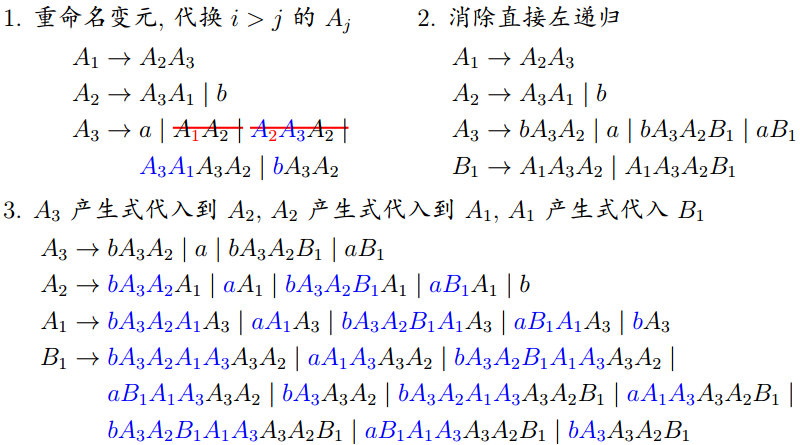6 下推自动机
6.1 下推自动机
形式定义：PDA，P为七元组

P

=

(

Q

,

Σ

,

Γ

,

δ

,

q

0

,

Z

0

,

F

)

P=(Q,\Sigma,\Gamma,\delta,q_0,Z_0,F)

Q，有穷状态集

Σ

\Sigma

，有穷输入符号集

Γ

\Gamma

，有穷栈符号集

δ

\delta

：

Q

×

(

Σ

∪

{

ϵ

}

)

×

Γ

→

2

Q

×

Γ

∗

Q\times (\Sigma\cup\{\epsilon\})\times\Gamma\rightarrow 2^{Q\times\Gamma^*}

，状态转移函数

q

0

∈

Q

q_0\in Q

，初始状态

Z

0

∈

Γ

−

Σ

Z_0\in\Gamma-\Sigma

，栈底符号

F

⊂

Q

F\subset Q

，接收状态集或终态集
本质上是带有栈的

ϵ

−

\epsilon-

NFA
举例：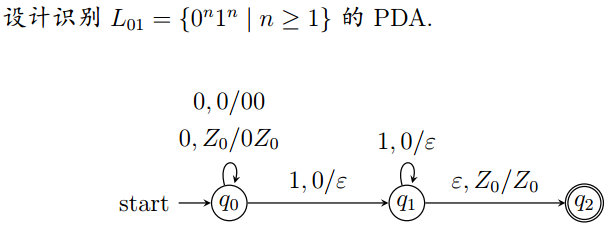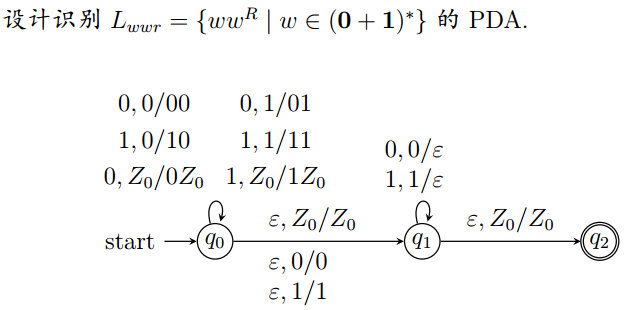瞬时描述：为描述 PDA 瞬间的格局, 定义

Q

×

Σ

∗

×

Γ

∗

Q\times\Sigma^*\times\Gamma^*

中三元组

(

q

,

ω

,

γ

)

(q,\omega,\gamma)

为瞬时描述（ID），表示此时PDA处于状态q，剩余输入串

ω

\omega

，栈为

γ

\gamma

转移符号：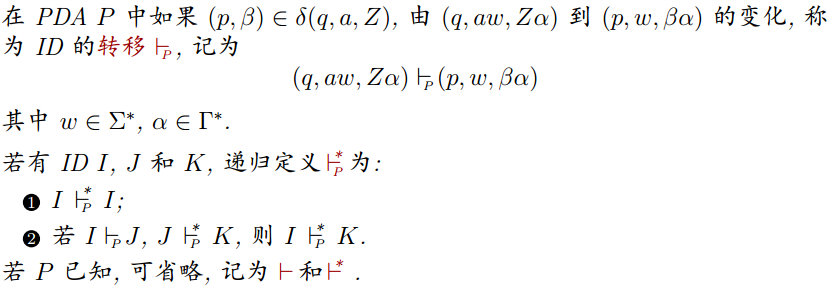对 PDA P 的一个合法 ID 序列 (计算)：
把相同的字符串加到所有ID的输入串末尾, 所得到的计算合法把相同的栈符号串加到所有ID的栈底之下, 所得到的计算合法把所有ID中都未消耗的部分输入串去掉, 所得到的计算合法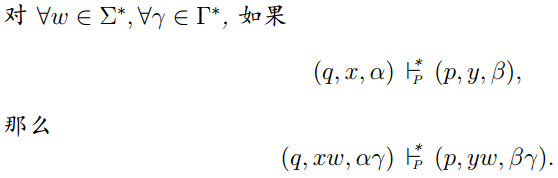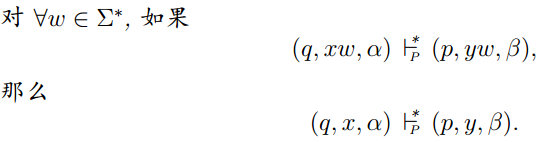6.2 下推自动机接受的语言
PDA

P

=

(

Q

,

Σ

,

Γ

,

δ

,

q

0

,

Z

0

,

F

)

P=(Q,\Sigma,\Gamma,\delta,q_0,Z_0,F)

以两种方式接受语言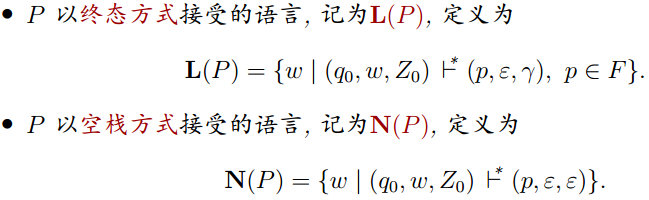如：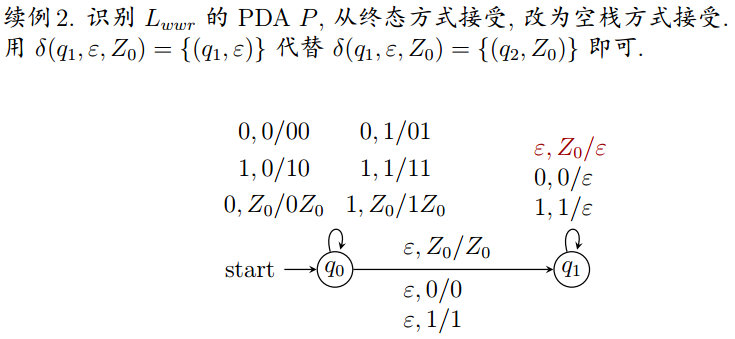此种方式无栈底符号
从终态方式到空栈方式：如果PDA $P_F$以终态方式接受语言L，则存在PDA $P_N$以空栈方式接受L
从空栈方式到终态方式：如果PDA $P_N$以空栈方式接受语言L，则存在PDA $P_F$以终态方式接受L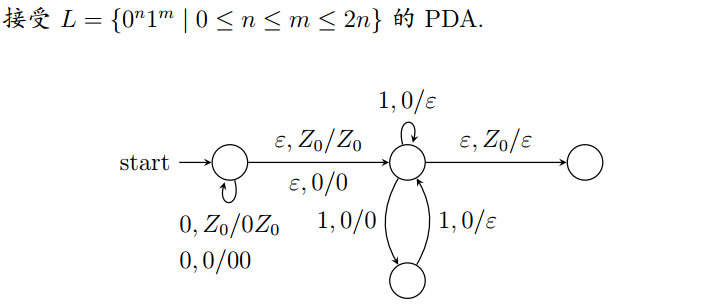6.3 下推自动机与文法的等价性
PDA到CFG：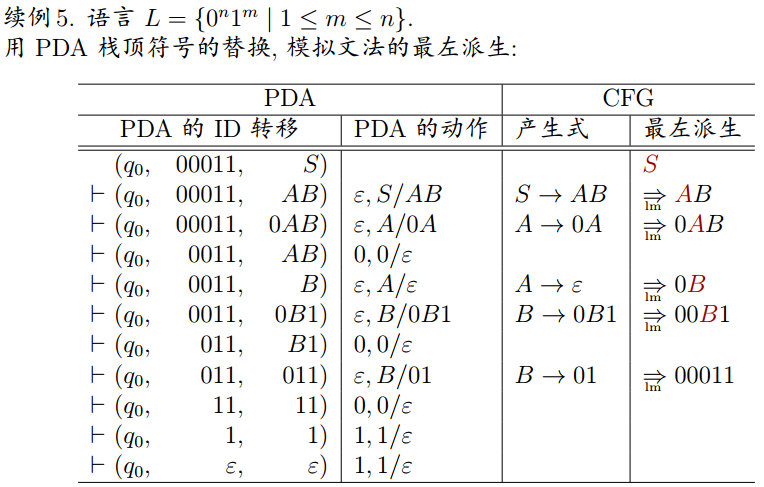构造与文法等价的 PDA：
如果CFG G = (V, T, P′, S)，构造PDA

P

=

(

{

q

}

,

T

,

V

∪

T

,

δ

,

q

,

S

,

∅

)

P=(\{q\},T,V\cup T,\delta,q,S,\emptyset)

其中，

δ

\delta

为

∀

A

∈

V

\forall A\in V

：

δ

(

q

,

ϵ

,

A

)

=

{

(

q

,

β

)

∣

A

→

β

∈

P

′

}

\delta(q,\epsilon,A)=\{(q,\beta)|A\rightarrow\beta\in P'\}

∀

a

∈

T

\forall a\in T

：

δ

(

q

,

a

,

a

)

=

{

(

q

,

ϵ

)

}

\delta(q,a,a)=\{(q,\epsilon)\}

那么L(G)=N(G)
举例：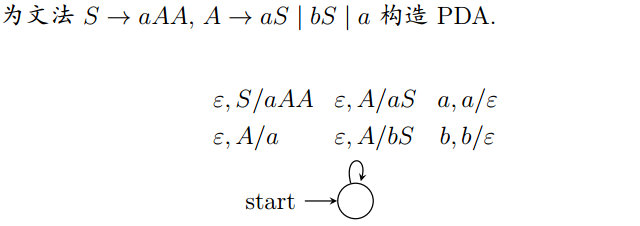构造与 GNF 格式文法等价的 PDA：
如果 GNF 格式的 CFG G = (V, T, P′, S), 那么构造 PDA

P

=

(

{

q

}

,

T

,

V

,

δ

,

q

,

S

,

∅

)

P=(\{q\}, T, V, \delta, q, S, \emptyset)

为每个产生式, 定义

δ

\delta

为:

δ

(

q

,

a

,

A

)

=

{

(

q

,

β

)

∣

A

→

a

β

∈

P

′

}

\delta(q,a,A)=\{(q,\beta)|A\rightarrow a\beta\in P'\}

举例：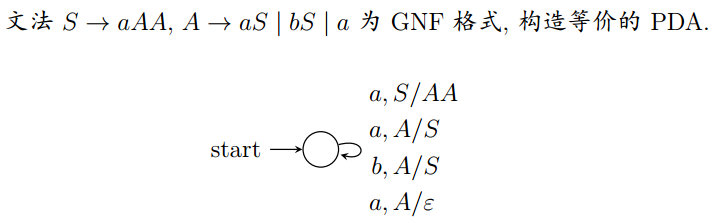由 PDA 到 CFG：
如果 PDA P , 有 L = N§, 那么 L 是上下文无关语言。
构造与 PDA 等价的 CFG：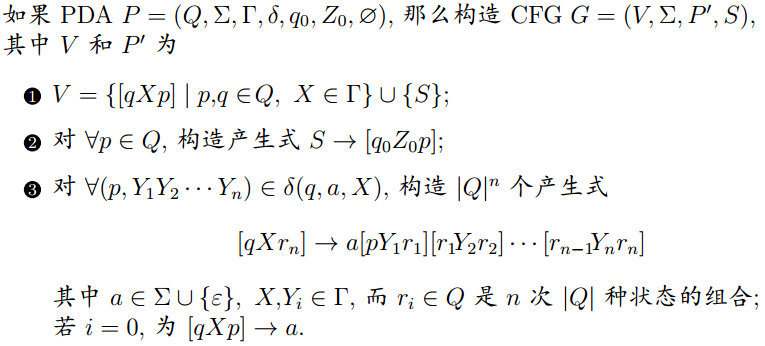6.4 确定性下推自动机
如果 PDA P=(

Q

,

Σ

,

Γ

,

δ

,

q

0

,

Z

0

,

F

Q, \Sigma, \Gamma, \delta, q_0, Z_0, F

) 满足

∀

a

∈

Σ

∪

{

ϵ

}

，

δ

(

q

,

a

,

X

)

\forall a\in\Sigma\cup\{\epsilon\}，\delta(q,a,X)

至多有一个动作

∃

a

∈

Σ

\exists a\in\Sigma

，如果

δ

(

q

,

a

,

X

)

≠

∅

\delta(q,a,X)\not=\emptyset

，那么

δ

(

q

,

ϵ

,

X

)

=

∅

\delta(q,\epsilon,X)=\emptyset

则称P为确定性下推自动机（DPDA）
DPDA P以终态方式接受的语言L§称为DCFL
DPDA与PDA不等价
正则语言与 DPDA：
定理：如果 L 是正则语言, 那么存在 DPDA P 以终态方式接受 L, 即 L = L§
定义：如果语言 L 中不存在两个不同的字符串 x 和 y，使 x 是 y 的前缀，称语言 L 满足前缀性质
定理：如果有DPDA P且L=N§，当且仅当L有前缀性质且存在DPDA P′使 L=L(P′)
DPDA 与无歧义文法
定理：DPDA P，语言 L = N§，那么 L 有无歧义的 CFG
定理：DPDA P，语言 L = L§，那么 L 有无歧义的 CFG
7 上下文无关语言的性质
7.1 上下文无关语言的泵引理
任何

Σ

\Sigma

上的所有语言是不可数的
任何

Σ

\Sigma

上的所有CFL是可数的
语法分析树的大小:
对于乔姆斯基范式文法 G = (V, T, P, S) 的语法树，如果产物为终结符串

ω

\omega

，且树中最长路径的长度是n，那么

∣

ω

∣

≤

2

n

−

1

|\omega|\le 2^{n-1}

上下文无关语言的泵引理：
如果语言L时CFL，那么存在正整数N，它只依赖于L，对

∀

z

∈

L

\forall z\in L

，只要

∣

z

∣

≥

N

|z|\ge N

，就可以将z分成五部分

z

=

u

v

w

x

y

z=uvwxy

，满足

v

x

≠

ϵ

vx\not=\epsilon

（或

∣

v

x

∣

>

0

|vx|>0

）

∣

v

w

x

∣

≤

N

|vwx|\le N

∀

i

≥

0

,

u

v

i

w

x

i

y

∈

L

\forall i\ge 0,uv^iwx^iy\in L

举例：证明L=

{

0

n

1

n

2

n

∣

n

≥

1

}

\{0^n1^n2^n|n\ge 1\}

不是上下文无关语言
假设L是CFL，那么存在整数N，对

∀

z

∈

L

(

∣

z

∣

≥

N

)

\forall z\in L(|z|\ge N)

满足泵引理从L中去

z

=

0

N

1

N

2

N

z=0^N1^N2^N

，则显然

z

∈

L

z\in L

且

∣

z

∣

=

3

N

≥

N

|z|=3N\ge N

由泵引理，

z

z

可被分为

z

=

u

v

w

x

y

z=uvwxy

，且有

∣

v

w

x

∣

≤

N

|vwx|\le N

和

v

x

≠

ϵ

vx\not=\epsilon

那么vwx只能包含一种或两种字符：
一种字符, 或为 0, 或为 1, 或为 2, 那么

u

w

y

∉

L

uwy\not\in L

两种字符, 或为 0 和 1, 或为 1 和 2, 那么也有

u

w

y

∉

L

uwy\not\in L

与泵引理

u

w

y

=

u

v

0

w

x

0

y

∈

L

uwy=uv^0wx^0y\in L

矛盾，假设不成立L不是上下文无关的
举例：证明

L

=

{

w

w

∣

w

∈

{

0

,

1

}

∗

}

L=\{ww|w\in\{0,1\}^*\}

不是上下文无关的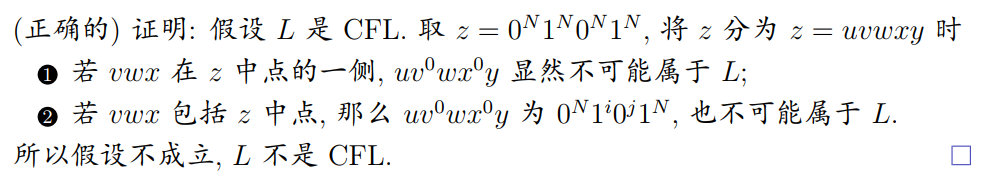7.2 上下文无关语言的封闭性
代换：两个字母表 Σ 到 Γ 的函数$s : \Sigma\rightarrow 2^{\Gamma∗} 称 为 代 换 ， 称为代换， \Sigma 中 的 一 个 字 符 a 在 s 的 作 用 下 为 中的一个字符a在s的作用下为 \Gamma 上 的 一 个 语 言 L a ， 即 上的一个语言 La，即 s(a)=L_a ， 扩 展 s 的 定 义 到 字 符 串 ， ，扩展s的定义到字符串， s(\epsilon)={\epsilon}，s(xa)=s(x)s(a) ， 再 扩 展 s 到 语 言 ， 对 ，再扩展s到语言，对 \forall L\subset\Sigma^* ， ， s(L)=\cup_{x\in L}s(x)$
上下文无关语言在并、连接、闭包、正闭包、同态、反转、代换、逆同态下封闭。
CFL 对交/补运算不封闭
若 L 是 CFL 且 R 是正则语言, 则 L

∩

\cap

R 是 CFL
应用举例：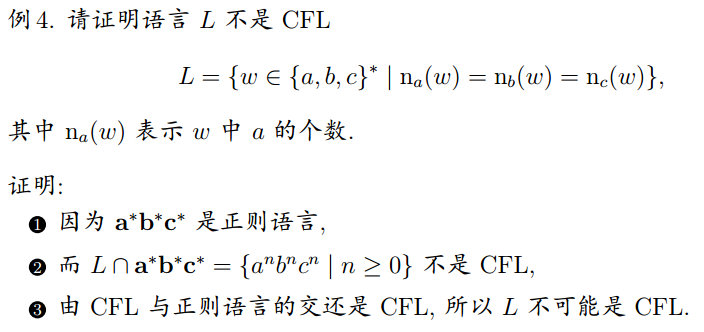7.3 上下文无关语言的判定性质
可判定的 CFL 问题：
空性: 只需判断文法的开始符号S是否为非产生的有穷性和无穷性
用不带无用符号的CNF的产生式画有向图变元为顶点, 若有A$\rightarrow$BC，则A到B和C各画一条有向边检查图中是否有循环 成员性：利用CNF范式，有CYK算法检查串w是否属于L
CYK算法：
CNF G=(V, T, P, S)，以O(

n

3

n^3

)时间检查"

w

=

a

1

a

2

…

a

n

∈

L

(

G

)

w=a_1a_2…a_n\in L(G)

？"
以动态规划的方式，在表中由下至上逐行计算

X

i

j

X_{ij}

，再检查"

S

∈

X

1

n

S\in X_{1n}

？"
计算首行

X

i

i

=

{

A

∣

A

→

a

i

∈

P

}

X_{ii}=\{A|A\rightarrow a_i\in P\}

计算其他

X

i

j

=

{

A

∣

i

≤

k

<

j

,

B

C

∈

X

i

k

X

k

+

1

,

j

,

A

→

B

C

∈

P

}

X_{ij}=\{A|i\le k<j,BC\in X_{ik}X_{k+1,j},A\rightarrow BC\in P\}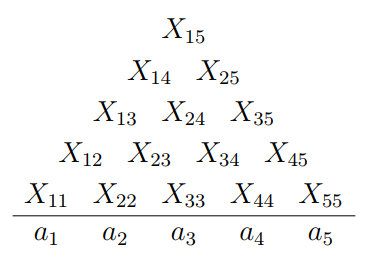举例：CNF G 如下, 用 CYK 算法判断 bbabaa

∈

\in

L(G)?

S

→

A

B

∣

B

C

A

→

B

A

∣

a

B

→

C

C

∣

b

C

→

A

B

∣

a

S\rightarrow AB|BC\\A\rightarrow BA|a\\B\rightarrow CC|b\\C\rightarrow AB|a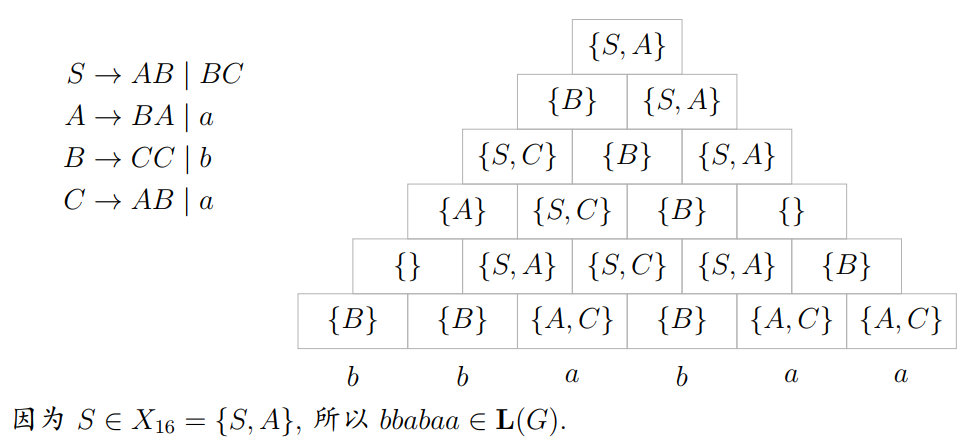7.4 乔姆斯基文法体系
如果文法 G = (V, T, P, S)，符号串

α

∈

(

V

∪

T

)

∗

V

(

V

∪

T

)

∗

,

β

∈

(

V

∈

T

)

∗

\alpha\in (V\cup T)^∗V (V\cup T)^∗,\beta\in (V\in T)^∗

,产生式都形如

α

→

β

\alpha\rightarrow\beta

，即每个产生式的左部

α

\alpha

中至少要有一个变元，那么
称G为0型文法或短语结构文法若

∣

β

∣

≥

∣

α

∣

|\beta|\ge |\alpha|

，称G为1型文法或上下文有关文法若

α

∈

V

\alpha\in V

，称G为2型文法或上下文无关文法若

α

→

β

\alpha\rightarrow\beta

都形如

A

→

a

B

A\rightarrow aB

或

A

→

a

A\rightarrow a

，称G为3型文法或正则文法
8 图灵机
8.1 图灵机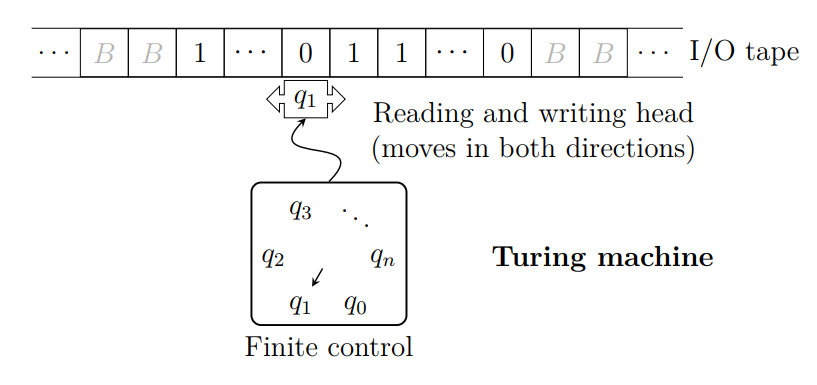图灵机M为七元组

M

=

(

Q

,

Σ

,

Γ

,

δ

,

q

0

,

B

,

F

)

M=(Q,\Sigma,\Gamma,\delta,q_0,B,F)

Q：有穷状态集

Σ

\Sigma

：有穷输入符号集

Γ

\Gamma

：有穷带符号集，且总有

Σ

⊂

Γ

\Sigma\subset\Gamma

δ

\delta

：

Q

×

Γ

→

Q

×

Γ

×

{

L

,

R

}

Q\times\Gamma\rightarrow Q\times\Gamma\times\{L,R\}

q

0

∈

Q

q_0\in Q

：初始状态

B

∈

Γ

−

Σ

B\in\Gamma-\Sigma

：空格符号

F

⊂

Q

F\subset Q

：终态集或接受状态集
图灵机的动作及状态转移图：
有穷控制器处于状态 q，带头所在单元格为符号 X，如果动作的定义为

δ

(

q

,

X

)

=

(

p

,

Y

,

L

)

\delta(q,X)=(p,Y,L)

，表示状态转移到了p，单元格改为Y，然后将带头向左移动一个单元格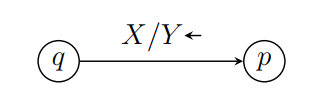举例：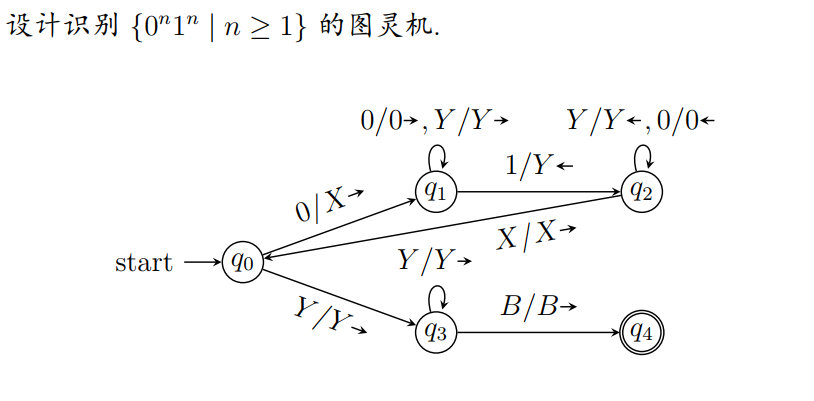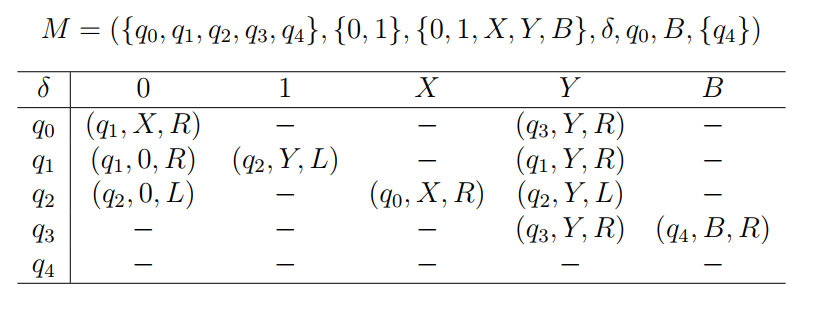瞬时描述：
图灵机虽有无穷长的带, 但非空内容总是有限的. 因此用带上全部的非空符号、当前状态和带头位置, 同时定义瞬时描述（ID）为：

X

1

X

2

.

.

.

X

i

−

1

q

X

i

X

i

+

1

.

.

.

X

n

X_1X_2...X_{i-1}qX_iX_{i+1}...X_n

图灵机当前状态q带头在左起第i个非空格符上

X

1

X

2

…

X

n

X_1X_2…X_n

是最左到最右非空格内容为避免混淆, 一般假定Q和

Γ

\Gamma

不相交
转移符号：
如：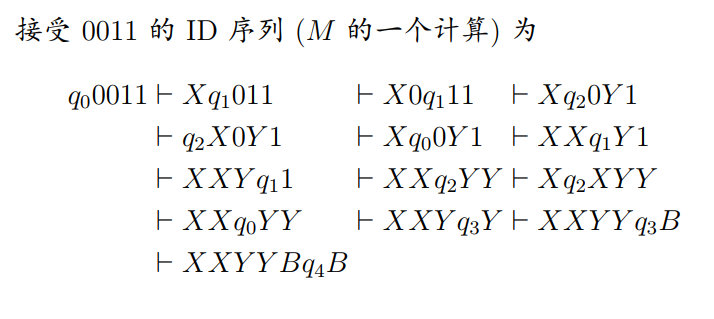图灵机的语言：
如果

M

=

(

Q

,

Σ

,

Γ

,

δ

,

q

0

,

B

,

F

)

M=(Q,\Sigma,\Gamma,\delta,q_0,B,F)

是一个图灵机, 则 M 接受的语言为

L

(

M

)

=

{

ω

∣

ω

∈

Σ

∗

,

q

0

ω

⊢

∗

α

p

β

,

p

∈

F

,

α

,

β

∈

Γ

∗

}

L(M)=\{\omega|\omega\in\Sigma^*,q_0\omega\vdash^*\alpha p\beta,p\in F,\alpha,\beta\in\Gamma^*\}

如果 L 是图灵机 M 的语言, 即 L = L(M), 则称 L 是递归可枚举语言
对接受和不接受的输入, 都保证停机的图灵机, 所接受的语言称为递归语言
如果$f(i_1, i_2, . . . , i_k) 对 所 有 对所有 i_1, i_2, . . . , i_k 都 有 定 义 , 称 都有定义, 称 f 为 全 递 归 函 数 ， 被 图 灵 机 计 算 的 函 数 为全递归函数，被图灵机计算的函数 f(i_1, i_2, . . . , i_k)$称作部分递归函数
展开全文• 哈尔滨工业大学形式语言课件与作业 其中有自动机理论与形式语言的学习
• 因为属于疫情期间，所以软件构造、形式语言与自动机难度较低，软件构造的复习一定注意设计模式的记忆以及英文专有名词的记忆。算法考试堪称史上最难，大家参考一下就好，算法考试要想高分还是要平时多练习昂。 ...
• 形式化定义1. 瞬时描述2. 停机2. 构造3. 双栈自动机 (Two Stack Machine)4. 图灵机编码1. 字符串排序枚举2. 编码5. TM接受的语言1. 递归可枚举语言2. 非递归可枚举语言3. 递归语言4. 通用语言5. 语言的范畴6. ...
• 文章目录：正则表达式1....ε\varepsilonε 是一个正则表达式，表示语言 {ε}\{\varepsilon\}{ε} ; ϕ\phiϕ 是一个正则表达式，表示空语言 ϕ\phiϕ ; ∀a∈Σ\forall a \in \Sigma∀a∈Σ，aaa 是一个正则表达式
• 形式化定义 2. 构造 3. 正则语言 2. 非确定的有穷自动机 (NFA) 3. DFA和NFA的等价性 4. ε-NFA和最小化DFA 1. ε-NFA的形式化定义 2. ε-闭包 3. DFA的最小化问题 1. 确定的有穷自动机 (DFA) 1. 形式化定义 确定的...
• 形式化定义 2. 语法分析树 3. 二义性 4. CFG的化简 5. 乔姆斯基范式(CNF) 2. 下推自动机(PDA) 1. 形式化定义 2. 确定的PDA 3. PDA的瞬时描述 4. PDA接受的语言 3. CFG和PDA的等价性 1. CFG ⇒\Rightarrow⇒ PDA 2. ...
• 不要浪费时间了，你要的我这里全打包好了，内含课后题答案详解+精炼讲义，是非常实用的素材，因为学习的时候感觉自己因为资料不足而很耗费精力，所以发上来帮助学弟学妹摆脱抽象的烦恼。
• 形式语言与自动机第一章答案~~~~~~~~~~~~~~~~~~~~~~~~~~~~~~~~~~~~~~~~~~~~~~~~~~~~~~~~~
• 包含形式语言与自动机理论ppt，1718级考试题和大作业，适合哈工大的小伙伴们食用。私聊我还可以得到额外福利（大作业）
• 接下来，小编为帮助报考哈尔滨工业大学2021考研考生，快速知晓该校2021考研复试要求等信息，特意整理出——2021考研复试:哈尔滨工业大学计算学部复试参考，供考生参考。2021考研复试:哈尔滨工业大学计算学部复试参考......